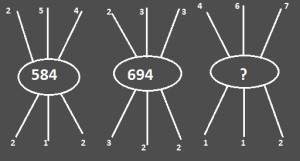Home > Math Shortcuts > Brain Trigger #18 – Sample Number puzzle question

# Brain Trigger #18 – Sample Number puzzle question

Question answers on Number puzzle which are common in competitive exams will be discussed here in this section. These types of Number puzzle questions will help you to prepare for your exam. Try to solve the question mention below using your skills on math. You can use shortcut methods to solve these Number puzzle questions.

Every types of Number puzzle problems and its shortcut tricks, how to do methods, you will find here in this website. what you need to do is to apply these tricks in Number puzzle problem to solve. However it’s possible that you may not need any tricks to solve few questions.

Now here we will give you a question on Number puzzle to solve. All you need to do is to read the question very carefully and try to solve it by yourself. Answer will be provided below. If you solve the question then check your answer with our solution. If you don’t know how to do it then check the solution below.

Each page contains a question on Number puzzle and also its solution with explanation. Click on the Next/Previous link to navigate through other questions.

Let’s begin the question and answer session. Today’s question is:

What number should replace the question mark ?Scroll down below to know the answer of this question. You can take as much time as you want to answer the problem. But always try to do it as faster as you can.

(Scroll down to get the answer)

(Scroll down to get the answer)

(Scroll down to get the answer)

(Scroll down to get the answer)

Now here we will discuss the answer of previous question. If you already try the problem and got an solution then check your answer with our solution. Well Done if you correctly answer the previous question, but if you are not able to answer it correctly then don’t worry. Solution with explanation of the following question is provided below.

You can solv this puzzle in this way…

firts pic- from top left number multiply with lower right number 2 x 2 = 4
from top right number multiply with lower left number 4 x 2 = 8
from top middle number multiply with lower middle number 5 x 1 = 5
So ans is 584

Similarly second would be same.. 2 x 2 = 4, 3 x 3 = 9, 3 x 2 = 6 = 694
Similarly third would be same…….4 x2 = 8, 7 x 1 = 7, 6 x 1 = 6 = 678